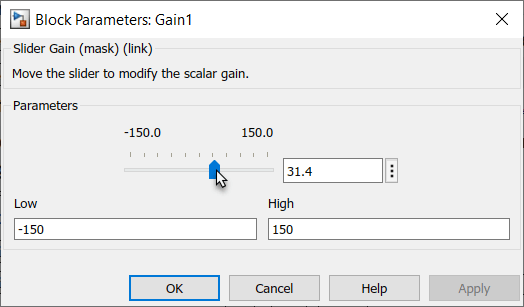Main Content

# Model a Redundant Sensor Pair by Using Atomic Subcharts

This model shows how to model a redundant pair of sensors. By using atomic subcharts, you can compose a large Stateflow® chart from other charts that reside in a library file.### Main Chart

In this model, the chart RedundantSensors contains two linked atomic subcharts (`Sensor1` and `Sensor2`) and a state (`Alarm`).At the start of the simulation, the subchart `Sensor1` is active. This atomic subchart reads the input `u1`. If the input value remains between 75 and -75, `Sensor1` passes this value as the output of `y`. Otherwise, the sensor fails and subchart `Sensor2` becomes active.

In a similar way, `Sensor2` reads the input `u2` and checks that its value remains between 100 and -100. `Sensor2` passes this value as the output of `y`. Otherwise, the sensor fails and the chart transitions to the `Alarm` state.

### Library Chart

The logic for both `Sensor1` and `Sensor2` is defined in a library model. In this model, the chart SingleSensor accepts an input `u` and provides a filtered sensor output `y`.The chart detects out-of-range errors in the sensor input `u`. Initially, the sensor is in the state `OK`. If `u` goes out of range, the chart takes the transition from `OK` to the state `FailOnce`. If `u` stays out of range for longer than one second, then the chart transitions to the state `Fail`. In this case, the sensor outputs a constant value of zero. This pattern allows the sensor to ignore transient spikes in the sensor reading.

### Map Inputs, Outputs, and Parameters

The chart RedundantSensors has two inputs (`u1` and `u2`), while the library chart that defines the atomic subcharts has only one input (`u`). To enable the atomic subcharts to access a different chart input, right-click each subchart and select Subchart Mappings. In the Mappings tab of the properties dialog box, you can:

• Specify which symbol in the main chart corresponds to each symbol in the subchart.

• Assign values to parameters defined in the subchart.

For example, in the case of subchart `Sensor1`:

• The subchart input `u` is mapped to the main chart input `u1`.

• The subchart output `u` is mapped to the main chart output `y`.

• The subchart parameters `HIGH` and `LOW` are assigned the values 75 and -75.In the case of subchart `Sensor2`:

• The subchart input `u` is mapped to the main chart input `u2`.

• The subchart output `u` is mapped to the main chart output `y`.

• The subchart parameters `HIGH` and `LOW` are assigned the values 100 and -100.

### Simulation Behavior

Initially, both inputs and both outputs to the chart are zero. To change the value of the chart inputs, double-click the Gain blocks and drag the slider.As long as the value of `u1` is between -75 and 75, the output value `y` tracks the input value `u1`. If the value of `u1` exceeds these bounds, the value of `y` begins to track the input value `u2`. If the value of `u2` falls outside the range from -100 to 100, `y` returns a value of zero and `Alarm` returns a value of one.

## SupportGet trial now